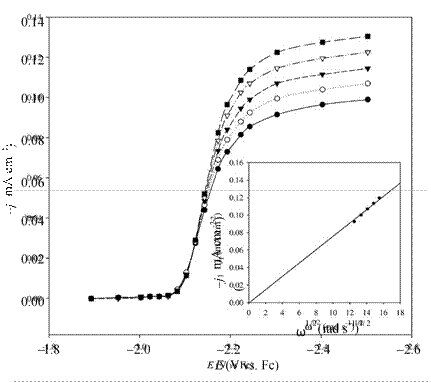# Electrochemistry of Room-Temperature/ Articles about substances designated as ionic liquids have begun to appear with increasing regularity in chemistry journals around the world, страница 14

Epa + Epc

E1/2 =(9)

2

E1/2 is related to the formal potential, E0', of the redox reaction by the following expression

1/ 2E = E 0' + 2.303RT log⎛⎜DR ⎞⎟                               (10)

1/ 2                          ⎜DO ⎟⎠ nF

where DR and DO are the diffusion coefficients for the reduced and oxidized species, respectively.

The chemical stability of the reduction or oxidation product produced during the initial voltammetric scan and/or the presence of homogeneous reactions coupled to the charge transfer process can often be determined by examining the appropriate peakcurrent ratio as a function of scan rate.336 For a cathodic process, the ratio of interest is ipa / ipc, where ipa and ipc are the absolute magnitudes of the anodic and cathodic peak currents, respectively. For an anodic reaction, the ratio ipc / ipa provides the desired information. Because the peak current obtained on the reverse scan, whether ipa or ipc, depends strongly on the switching potential for the initial scan, Eλ, there is no simple graphical procedure that can be used to obtain this current. However, as noted by Nicholson,337 the peak current ratio for a reversible reaction can be expressed a function of three parameters in the following empirical relationship for a cathodic reaction

ipa        (ipa )0       0.485(isp )0= + + 0.086  (11) ipc ipc ipcFigure 7. Cyclic staircase voltammograms recorded at different sweep rates at a platinum disk electrode in EtMeIm+F(HF)2.3- containing 11.83 mmol L-1 ferrocene.326  (inset) ratio of the cathodic to anodic peak currents as a function of sweep rate for the voltammograms shown in this figure.

where (ipa)0 and (isp)0 are the magnitudes of the anodic current and the current at Eλ, respectively, measured with respect to the baseline for the cathodic current. The corresponding equation for ipc/ipa is then

ipc        (ipc )0      0.485(isp )0= + + 0.086  (12) ipa ipa ipa

where (ipc)0 is the absolute magnitude of the cathodic current measured with respect to the baseline for the anodic current. Figure 7 shows a series of CSVs recorded for the oxidation of ferrocene [Fe(cp)2] in EtMeIm+F (HF)2.3 at different scan rates and the corresponding plots of  ipc/ipa as a function of scan rate, which was estimated by  using Eq. (12).326  The oxidation  of Fe(cp)2 in thisFigure 8. Voltammograms recorded as a function of angular velocity, ω, at a mercury film rotating disk electrode in n-Bu3MeN+Tf2N- containing 27.8 mmol L-1 Cs(I).  (inset) relationship between the limiting current density, jl, and ω1/2. Reproduced from Ref. 150 with permission of Elsevier Ltd.

solvent is known to be electrochemically and chemically reversible, and as expected, ipc/ipa is close to unity and essentially independent of the scan rate. For a complete discussion of the effects of coupled homogeneous chemistry on the peak current ratio, the original article by Nicholson and Shain336 should be consulted. It is the experience of the authors that Eqs. (11) and (12) can also be qualitatively applied to most quasi-reversible systems with reasonably good results.

## (ii) Hydrodynamic Voltammetry

The most common hydrodynamic technique employed for voltammetric measurements is that based on rotating electrodes, mainly rotating disk electrodes (RDE) and rotating ring-disk electrodes (RRDE). The main benefit of hydrodynamic or convectivediffusion voltammetry is that the rate of mass transport of the electroactive species to the electrode surface can be precisely controlled by adjusting the rotation rate of the electrode. Because the electrode potential is scanned very slowly, there is no measurable current contribution from double-layer charging. Changing the electrode rotation rate in RDE voltammetry is analogous to changing the scan rate in stationary electrode voltammetry. Figure 8 shows a voltammogram recorded as a function of angular velocity at a mercury film RDE in n-Bu3MeN+Tf2N- containing Cs+.150 Under mass-transport-limited conditions, the absolute magnitude of the limiting current, il, should always vary linearly with the square root of the electrode rotation rate, ω, according to the Levich equation: